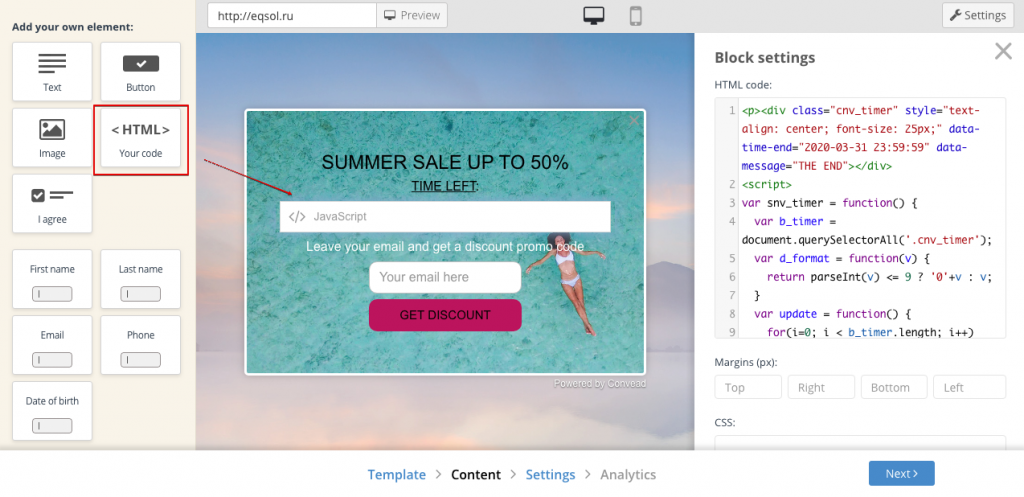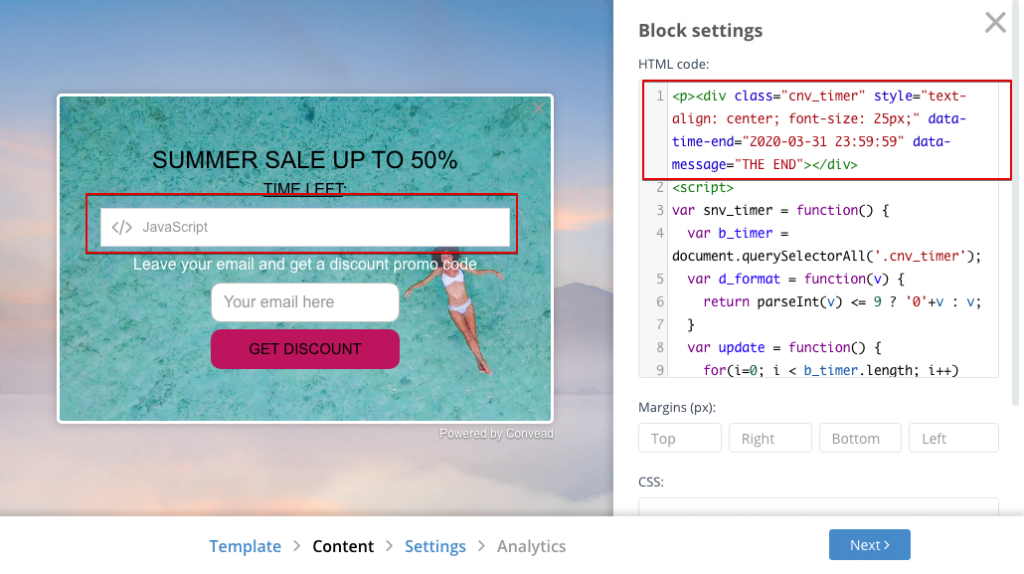# How to insert a timer element into a widget

Let’s look at how you can make a countdown timer widget in Convead. It may look something like this:To create such a timer, you need to add an HTML block to the widget:In this block you need to insert the code, depending on what timer you want. Most often our clients use one of two timers:

1. A single timer for all visitors for a few days during which some promotion is in operation. Or “Until New Year … X days remaining”.

2. A personal timer for each visitor showing the period when they can use their promotional code.

Below are the codes for both options:

Single-timer HTML code for a specific date

This code specifies the date until which the countdown timer is displayed. When the date arrives, the text “Promotion completed” is displayed. Copy the timer code and paste it into the HTML block:

``<code><div style="text-align: center; font-size: 20px;" data-time-end="2019-05-19 23:59:59" data-message="Promotion completed"></div> <script> var snv_timer = function() { var b_timer = document.querySelectorAll('.cnv_timer'); var d_format = function(v) { return parseInt(v) <= 9 ? '0'+v : v; } var update = function() { for(i=0; i < b_timer.length; i++) { var b_el = b_timer[i]; var time_end = new Date(b_el.dataset.timeEnd.replace(/(\d+)-(\d+)-(\d+)/, '\$2/\$3/\$1')); var time_real = (new Date()); if (time_end < time_real) { b_el.innerHTML = b_el.dataset.message; } else { var s = time_end.getTime() - time_real.getTime(); s = parseInt(s / 1000); var d = parseInt(s / 86400); s -= d * 86400; var h = parseInt(s / 3600); s -= h * 3600; var m = parseInt(s / 60); s -= m * 60; b_el.innerHTML = d + ' д. ' + d_format(h) + ':' + d_format(m) + ':' + d_format(s); } } setTimeout(function() { if (b_timer.length > 0) update(); }, 1000); } update(); } snv_timer(); </script>``

HTML code for a unique timer for each visitor

This timer will start counting down from 100 seconds each time it is opened. When 100 seconds have elapsed, the user will see “Promotion completed”. Copy the timer code and paste it into the HTML block:

``<code><div style="text-align: center; font-size: 20px;" data-seconds="100" data-message="Promotion completed"></div> <script> var snv_timer = function() { var b_timer = document.querySelectorAll('.cnv_timer'); var d_format = function(v) { return parseInt(v) <= 9 ? '0'+v : v; } var update = function() { for(i=0; i < b_timer.length; i++) { var b_el = b_timer[i]; if (!b_el.dataset.current_mseconds) b_el.dataset.current_mseconds = b_el.dataset.seconds * 1000; var time_end = b_el.dataset.current_mseconds; if (time_end < 0) { b_el.innerHTML = b_el.dataset.message; } else { var s = time_end; s = parseInt(s / 1000); var d = parseInt(s / 86400); s -= d * 86400; var h = parseInt(s / 3600); s -= h * 3600; var m = parseInt(s / 60); s -= m * 60; b_el.innerHTML = d_format(h) + ':' + d_format(m) + ':' + d_format(s); time_end = time_end - 1000; b_el.dataset.current_mseconds = time_end; } } setTimeout(function() { if (b_timer.length > 0) update(); }, 1000); } update(); } snv_timer(); </script>``

Do not worry that instead of the counter you see HTML code in the editor. Use the preview to see what the counter will look like.

### Timer settings

You can edit the date and time until which the widget will work, as well as adjust the size, color, and style of the text. This will require a basic knowledge of HTML and a firm hand. You can edit the text style, date, and end time of the timer at the top of the HTML code:You can make any timer similar to these examples. The only limitation here is your imagination!

Did this answer your question? Thanks for the feedback There was a problem submitting your feedback. Please try again later.